Disclaimer: This dissertation has been written by a student and is not an example of our professional work, which you can see examples of here.

Any opinions, findings, conclusions, or recommendations expressed in this dissertation are those of the authors and do not necessarily reflect the views of UKDiss.com.

# Learning Transaction Embedding via Items and Frequent Itemsets

Info: 7409 words (30 pages) Dissertation
Published: 25th Oct 2021

Tagged: Computer Science

## Abstract

Learning meaningful and effective representations for transaction data is a crucial prerequisite for transaction classification and clustering tasks. Traditional methods which use frequent itemsets (FIs) as features often suffer from the data sparsity and high-dimensionality problems. Several supervised methods based on discriminative FIs have been proposed to address these disadvantages, but they require transaction labels, thus rendering them inapplicable to real-world applications where labels are not given.

In this paper, we propose an unsupervised method which learns low-dimensional continuous vectors for transactions based on information of both singleton items and FIs. We demonstrate the superior performance of our proposed method in classifying transactions on four datasets compared with several state-of-the-art baselines.

## 1 Introduction

A transaction dataset consists of multiple transactions, each of which is a set of discrete and distinct items. It can be found in many different domains such as the products purchased in a supermarket basket and the symptoms diagnosed in a patient's admission. Turning such data into useful information and knowledge requires the applications of machine learning methods such as Support Vector Machine (SVM) or K-means. This task, however, is challenging because machine learning methods typically require inputs as fixed-length vectors, which are not applicable to transactions.

A common solution in data mining is to use frequent itemsets (FIs) as features . This method first mines FIs (i.e., itemsets whose supports (or frequencies) are not less than a minimum support threshold δ ) from the dataset. It then represents a transaction as a vector with binary components indicating whether this transaction contains a particular frequent itemset. Given a dataset D and the set of FIs discovered from D, F(D) = {X1,X2,...,XF}, the feature vector of a transaction T is defined as f(T) = [x1,x2,...,xF], where xi = 1 if XiT otherwise xi = 0. We can see that the dimension of the feature space is huge since the number of FIs is often very large. For example, on some datasets, the number of FIs is more than 105 with δ < 5%. Consequently, this leads to the high-dimensionality and data sparsity problems.

c Springer International Publishing AG, part of Springer Nature 2018 D. Phung et al. (Eds.): PAKDD 2018, LNAI 10939, pp. 361–372, 2018.

https://doi.org/10.1007/978-3-319-93040-4_29

To tackle these two disadvantages, many researchers have attempted to extract only significant FIs using discriminative measures such as support difference , support ratio , or information gain . However, all these measures require labels of transactions, making the mining process supervised. Due to the supervised nature of these methods, they have two limitations. First, their transaction representations are constructed for a particular mining task (e.g., transaction classification), thus the representations cannot be directly transferred to another task (e.g., transaction clustering). Second, the success of these methods relies on an enormous availability of labels for all training examples, a condition often not met in real applications.

## Our Approach

To overcome the weaknesses of FI-based methods and supervised FI-based methods, we propose a novel method for learning low-dimensional representations (also called embedding method) for transactions in a fully unsupervised fashion. In particular, our embedding method (named Trans2Vec) first represents a transaction using two different sets: a set of singleton items and a set of FIs. It then proposes two models to learn transaction embeddings: one learns embeddings from these two sets separately (individual-training model) and another learns embeddings from these two sets simultaneously (joint-training model). Trans2Vec owns two advantages. First, it is fully unsupervised. Compared to supervised FI-based methods, it can be directly used for learning transaction embeddings in domains where labeled examples are difficult to obtain. Moreover, the low-dimensional representations learned are well-generalized to many different tasks such as transaction classification and transaction clustering. Second, it leverages not only the information of singleton items but also that of FIs which have many benefits. Regarding , FIs are useful for constructing transaction features since (1) They can capture the associations among individual items; and (2) They can capture the relationships among transactions. In short, we make the following contributions:

1. We propose Trans2Vec, an unsupervised method, to learn low-dimensional continuous representations for transaction data.

2. We propose two models in Trans2Vec, which learn transaction embeddings from information of both singleton items and FIs. The embeddings learned are meaningful and discriminative.

3. We demonstrate Trans2Vec in transaction classification where it achieves significant improvements on several benchmark datasets.

## 2 Related Work

Our method is related to FI-based approaches. FIs have been used to construct feature vectors for transactions , which are essential inputs for many machine learning tasks such as transaction classification and clustering. However, this traditional approach suffers from the data sparsity and high-dimensionality problems due to the huge number of FIs discovered. To solve these two disadvantages, recently proposed methods have tried to extract significant and discriminative FIs only. For example, Cheng et al.  developed an approach which first mined FIs and then selected the most discriminative ones based on their information gain. Following the same procedure, discriminative FIs were discovered based on their support difference  and support ratio . Although discriminative FIs can help to reduce the feature space and are useful for classification, they require transaction labels, making the mining process supervised. Related to transaction classification, FIs have been also used to build rule-based classifiers, often called associative classification. These classifiers are constructed from highconfidence and high-support association rules which represent the strong associations between FIs and labels. A testing example is then predicted using one single rule  or multiple rules .

Our method is also related to embedding methods. Embedding learning has become a hot trend since 2013 when Mikolov introduced Word2Vec  to learn embedding vectors for words in text. In recent years, embedding methods have been developed to learn low-dimensional vectors for nodes in network , symptoms in healthcare , and documents in text [4,10]. As far as we know, learning embedding vectors for transactions has not been studied yet. In this paper, we propose the first method to learn transaction embeddings. Different from supervised FI-based methods and associative classification, our approach is fully unsupervised and leverages information of both items and FIs to learn transaction embeddings.

## 3 Framework

### 3.1 Problem Definition

We follow the notations in . Given a set of items I = {i1,i2,...,iM}, a transaction dataset D = {T1,T2,...,TN} is a set of transactions where each transaction Ti is a set of distinct items (i.e., Ti ⊆ I).

Our goal is to learn a mapping function f : D → Rd such that every transaction Ti ∈ D is mapped to a d-dimensional continuous vector. The mapping needs to capture the similarity among the transactions in D, in the sense that Ti and Tj are similar if f(Ti) and f(Tj) are close to each other on the vector space, and vice versa. The matrix X = [f(T1),f(T2),...,f(TN)] then contains feature vectors of transactions, which can be direct inputs for many traditional machine learning and data mining tasks, particularly classification.

### 3.2 Learning Transaction Embeddings Based on Items

We adapt the Paragraph Vector-Distributed Bag-of-Words (PV-DBOW) model introduced in  to learn embedding vectors for transactions, where each transaction is treated as a document and items are treated as words. Given a target transaction Tt whose representation needs to be learned, and a set of items I(Tt) = {i1,i2,...,ik} contained in Tt, our goal is to maximize the log probability of predicting the items i1,i2,...,ik which appear in Tt: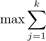logPr(ij | Tt) (1)

Furthermore, Pr(ij | Tt) is defined by a softmax function:

Pr(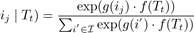, (2)

where g(ij) ∈ Rd and f(Tt) ∈ Rd are embedding vectors of the item ij and the transaction Tt respectively, and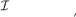is the set of all singleton items.

Computing the summation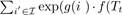)) in Eq.2 is very expensive since the number of items in I is often very large. To solve this problem, we approximate it using the negative sampling technique proposed in Word2Vec . The idea is that instead of iterating over all items in I, we randomly select a relatively small number of items which are not contained in the target transaction Tt (these items are called negative items). We then try to distinguish the items contained in Tt from the negative items by minimizing the following binary objective function of logistic regression: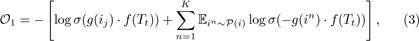where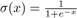is a sigmoid function, P(i) is the negative item collection, in is a negative item draw from P(i) for K times, and g(in) ∈ Rd is the embedding vector of in.

We minimize O1 in Eq.3 using stochastic gradient descent (SGD) where the gradients are derived as follows: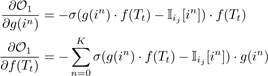, (4)

where Iij[in] is an indicator function to indicate whether in is an item ij (i.e., the negative item is contained in the target transaction Tt) and when n = 0, then in = ij.

### 3.3 Learning Transaction Embeddings Based on Frequent Itemsets

As discussed in Sect.1, FIs are more advantageous than singleton items since they can capture more information in transactions. We believe that if we learn transaction embeddings based on FIs instead of items, then the transaction representations learned are more meaningful and discriminative.

Following the notations in , we define a frequent itemset as follows. Given a set of items I = {i1,i2,...,iM} and a transaction dataset D = {T1,T2,...,TN}, an itemset X is a set of distinct items (i.e., X ⊆ I). The support of X is defined as sup(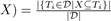, i.e., the fraction of transactions in D, which contain X. Given a minimum support threshold δ ∈ [0,1], X is called a frequent itemset if sup(X) ≥ δ.

Example 1. Consider an example transaction dataset with five transactions, as shown in Fig.1(a). Let δ = 0.6. The itemset {b,c} (or bc for short) is contained in three transactions T1, T2, and T4; thus, its support is sup(bc) = 3/5 = 0.6. We say that bc is a frequent itemset since sup(bc) ≥ δ. With δ = 0.6, there are in total six FIs discovered from the dataset, as shown in Fig.1(b), and each transaction now can be represented by a set of FIs, as shown in Fig.1(c).

 Trans Items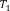{a, b, c}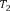{b, c, d}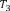{a, d}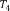{b, c, d, e}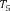{a, c, d}
 FI Items sup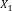{a} 0.6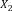{b} 0.6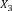{c} 0.8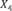{d} 0.8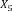{b, c} 0.6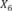{c, d} 0.6
 Trans FIs{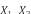,,}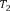{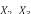,,,}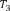{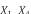}{,,,}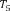{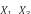,,}

(a) (b) (c)

Fig.1. Two forms of a transaction: a set of single items and a set of FIs. Table (a) shows a transaction dataset with five transactions where each of them is a set of items. Table (b) shows six FIs discovered from the dataset (here, δ = 0.6). Table (c) shows each transaction represented by a set of FIs.

Following the same procedure in Sect.3.2, given the set of FIs F(Tt) = {X1,X2,...,Xl} contained in the target transaction Tt, the objective function to learn the embedding vector for Tt based on its FIs is defined as follows: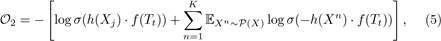where h(Xj) ∈ Rd is the embedding vector of the frequent itemset Xj ∈ F(Tt), P(X) is the negative frequent itemset collection (i.e., a small set of random FIs which are not contained in Tt), Xn is a negative frequent itemset drawn from P(X) for K times, and h(Xn) ∈ Rd is the embedding vector of Xn. We minimize O2 in Eq.5 using SGD.

### 3.4 Trans2Vec Method for Learning Transaction Embeddings

When learning an embedding vector for a transaction Tt based on its FIs, there is a possible situation that Tt does not contain any FIs. In this case, we cannot learn a useful embedding vector; instead, we simply use a zero vector with the size of d (i.e., f(Tt) = [0,0,...,0]). To avoid this problem, we propose two models which combine information of both items and FIs to learn embedding vectors for transactions. These two models named individual-training and joint-training are presented next.

## Individual-Training Model to Learn Transaction Embeddings

The basic idea, as illustrated in Fig.2, is that given a transaction Tt, we learn an embedding vector f1(Tt) for Tt based on its items (see Sect.3.2) and an embedding vector f2(Tt) for Tt based on its FIs (see Sect.3.3). We then take the average of two embedding vectors to obtain the final embedding vector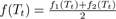for that transaction.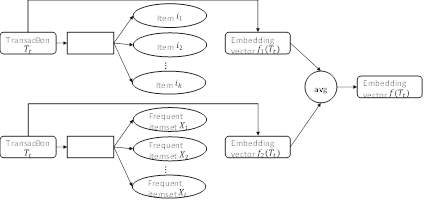Fig.2. Individual-training model. Given a transaction Tt, we learn the embedding vectors f1(Tt) and f2(Tt) based on its items and FIs, respectively. We then take the average of f1(Tt) and f2(Tt) to obtain the final embedding vector f(Tt).

## Joint-Training Model to Learn Transaction Embeddings

In the individual-training model, the relationships between items and FIs are not considered since they are used independently. Consequently, the transaction embeddings only capture the latent relationships between transactions and items and those between transactions and FIs separately. To tackle this weakness, we further propose the joint-training model which uses information of both items and FIs of a transaction simultaneously. The overview of this model is shown in Fig.3. Specifically, given a transaction Tt, our goal is to minimize the following objective function: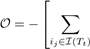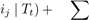logPr(logPr(Xj | Tt)⎦, (6)

Xj∈F(Tt)

where I(Tt) is the set of singleton items contained in Tt and F(Tt) is the set of FIs contained in Tt.

Equation6 can be simplified to: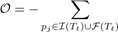logPr(pj | Tt), (7)

where pjTt is an item or a frequent itemset (in general, we call pj a pattern).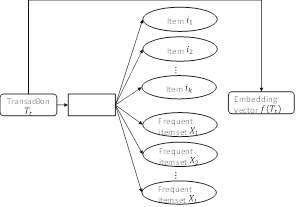Fig.3. Joint-training model. Given a transaction Tt, we learn the embedding vector f(Tt) for Tt based on both its items and FIs.

Following the same procedure in Sect.3.2, we minimize the following objective function: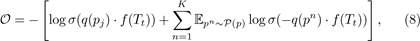where q(pj) ∈ Rd is the embedding vector of the pattern pj ∈ I(Tt) ∪ F(Tt), P(p) is the negative pattern collection (i.e., some random patterns which are not contained in Tt), pn is a negative pattern drawn from P(p) for K times, and q(pn) ∈ Rd is the embedding vector of pn.

We minimize Eq.8 using SGD. After the learning process is completed, the embedding vector f(Tt) is learned for the transaction Tt, and the embedding vectors of two transactions Ti and Tj are close to each other if they have similar items and FIs.

## 4 Experiments

We conduct extensive experiments on real-world transaction datasets to quantitatively evaluate the performance of Trans2Vec in transaction classification.

### 4.1 Datasets

We use four benchmark datasets whose characteristics are summarized in Table1. Snippets  consists of web search transactions where each of them is a set of keywords (e.g., "supplier", "export") and is classified into one of eight categories (e.g., "business"). Cancer  is a dataset of patient admissions where each admission is a list of diagnosed symptoms (e.g., "cough", "headache") and is labeled regarding the re-admission status of a patient. Retail  is a transaction dataset which contains the transactions occurring between 01/12/2010 and 09/12/2011 of a United Kingdom-based online retailer. Each transaction is a set of products purchased by customers from England or another country. Foodis a collection of food baskets, each of which is a list of foods (e.g., "milk") purchased by a customer and is labeled regarding whether the customer uses coupon.

Table 1. Statistics of four transaction datasets.

 Dataset # trans # train # test # items avg. length # classes Snippets 12,340 10,060 2,280 23,686 13.00 8 Cancer 15,000 12,000 3,000 3,234 6.00 3 Retail 3,000 2,400 600 3,376 26.93 2 Food 4,000 3,200 800 1,559 25.87 2

### 4.2 Baselines

For a comprehensive comparison, we employ six state-of-the-art up-to-date baselineswhich can be categorized into three main groups:

Natural Language Processing (NLP)-based methods: By treating a transaction as a document and items as words, we can apply methods in NLP to represent transactions. We select two well-known methods, namely Bag-ofWords (BOW) and Term Frequency-Inverse Document Frequency (TF-IDF).

FI-based methods: Given a dataset D and the set of FIs discovered from D, F(D) = {X1,X2,...,XF}, we employ two methods to represent transactions based on FIs. Given a transaction T, the first method (named FI-BIN) constructs the feature vector for T as f(T) = [x1,x2,...,xF] where xi = 1 if XiT otherwise xi = 0 while the second method (named FI-SUP) constructs the feature vector for T as f(T) = [x1,x2,...,xF] where xi = sup(Xi) if XiT otherwise xi = 0.

Embedding methods: We learn embedding vectors for transactions using two simple ways. The first method is based on items (see Sect.3.2), which we name TRANS-IT. The second method is based on FIs (see Sect.3.3), which we name TRANS-FI.

Our proposed method Trans2Vec has two different models which use different combinations of items and FIs. We denote Trans2Vec-IND for the model which learns transaction embeddings from items and FIs separately and then takes the average (see Sect.3.4) and denote Trans2Vec-JOI for the model which learns transaction embeddings from items and FIs simultaneously (see Sect.3.4).

### 4.3 Evaluation Metrics

Once the vector representations of transactions are constructed or learned, we feed them to an SVM with linear kernel  to classify the transaction labels. We use the linear-kernel SVM (a simple classifier) and do not tune the parameter C of SVM (here, we fix C = 1) since our focus is on the transaction embedding learning, not on a classifier. Each dataset is randomly shuffled and split into the training and test sets as shown in Table1. All methods are applied to the same training and test sets. We repeat the classification process on each dataset 10 times and report the average classification accuracy and the average F1-macro score. We do not report the standard deviation since all methods are very stable (their standard deviations are less than 10−2).

### 4.4 Parameter Settings

Our method Trans2Vec has two important parameters: the minimum support threshold δ for extracting FIs and the embedding dimension d for learning transaction embeddings. Since we develop Trans2Vec in a fully unsupervised learning fashion, the values for δ and d are assigned without using transaction labels. We set d = 128 (a common value used in embedding methods ) and set δ following the elbow method in . Figure4 illustrates the elbow method. From the figure, we can see when the δ value decreases, the number of FIs slightly increases until a δ value where it significantly increases. This δ value, highlighted in red in the figure and chosen by the elbow method without considering the transaction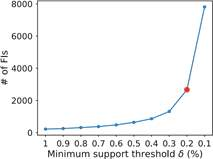Fig.4. The number of FIs discovered from the training set of Snippets dataset per δ. The δ value selected via the elbow method is indicated by the red dot. (Color figure online)

labels, is used in our experiments. In Sect.4.6, we analyze the potential impact of selecting two parameters δ and d on the classification performance.

For a fair comparison, we use the same δ for Trans2Vec and three baselines FI-BIN, FI-SUP, and TRANS-FI. We also set d = 128 for two baselines TRANSIT and TRANS-FI.

### 4.5 Results and Discussion

From Table2, we can see two models in our method Trans2Vec clearly results in better classification on all datasets compared with other baselines. Compared with NLP-based methods, Trans2Vec-JOI achieves 4–19% and 2–13% improvements in accuracy over BOW and TF-IDF, respectively. Similar improvements can be also observed when comparing with FI-based methods. On three datasets Snippets, Retail, and Food, Trans2Vec-JOI outperforms FI-BIN and FI-SUP by large margins (achieving 7–23% and 2–108% gains over FI-BIN and FI-SUP).

For most cases, embedding baselines (TRANS-IT and TRANS-FI) are better than NLP- and FI-based methods. Moreover, TRANS-FI always outperforms TRANS-IT. This demonstrates that learning transaction embeddings from FIs is more effective than learning transaction embeddings from items, as discussed in Sect.3.3. Two our models (Trans2Vec-IND and Trans2Vec-JOI) are always superior than two embedding baselines. This proves that our proposal to incorporate information of both singleton items and FIs into the transaction embedding learning is a better strategy than learning transaction embeddings from items or FIs only.

We also observe Trans2Vec-JOI produces better results than Trans2VecIND on all datasets. This verifies our intuition in Sect.3.4 that the transaction embeddings learned from items and FIs simultaneously are more meaningful and discriminative since they can capture different latent relationships of transactions simultaneously.

Table 2. Accuracy (AC) and F1-macro (F1) of our Trans2Vec and six baselines on four transaction datasets. Bold font marks the best performance in a column. The last row denotes the δ values used by our method for each dataset.

 Snippets Cancer Retail Food Method AC F1 AC F1 AC F1 AC F1 BOW 66.32 65.83 48.57 48.57 77.33 77.31 63.12 63.12 TF-IDF 70.26 69.52 49.43 49.43 81.67 81.56 64.50 64.49 FI-BIN 64.52 63.96 48.52 48.44 77.67 77.62 61.75 61.74 FI-SUP 37.94 32.19 47.30 46.54 80.33 79.20 71.00 70.03 TRANS-IT 75.23 74.79 49.35 49.32 81.17 81.07 65.95 65.81 TRANS-FI 77.88 77.41 50.03 49.92 82.07 81.90 69.14 68.95 Trans2Vec-IND 78.80 77.92 50.10 50.02 82.23 82.12 69.22 69.12 Trans2Vec-JOI 79.05 78.31 50.34 50.28 83.43 83.36 72.51 72.47 δ (%) 0.2% 0.2% 0.7% 0.2%

### 4.6 Parameter Sensitivity

We examine how the different choices of two parameters δ and d affect the classification performance of Trans2Vec-JOI on three datasets Snippets, Cancer, and Food. Figure5 shows the classification results as a function of one chosen parameter when another is set to its default value. From Fig.5(a), we can see the values for δ selected by the elbow method always lead to the best accuracy. This demonstrates that the elbow method is an effective way to choose δ for methods which use frequent patterns, the same finding was also mentioned in . Another observation is that on Cancer, δ is gain of relatively little relevant to the predictive task where our classification performance just slightly changes with different values for δ.

From Fig.5(b), we observe a first-increasing and then-decreasing accuracy line on two datasets Snippets and Food when d is increased whereas the classification performance shows an increasing trend with an increasing d on Cancer. This finding differs from those in document embedding methods, where the embedding dimension mostly shows a positive effect on document classification .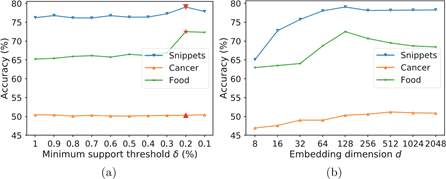Fig.5. Parameter sensitivity in transaction classification on the Snippets, Cancer, and Food datasets. The minimum support δ values selected via the elbow method and used in our experiments are indicated by red markers. (Color figure online)

## 5 Conclusion

We have presented Trans2Vec, an unsupervised method for learning transaction embeddings from information of both singleton items and FIs. Our comprehensive experiments on four transaction datasets demonstrated the meaningful and discriminative representations learned by our method in the transaction classification task. In particularly, Trans2Vec significantly outperforms several stateof-the-art baselines in both accuracy and F1-macro scores. One of our future work is to investigate the quality of our embeddings in the transaction clustering task. Another possible extension is to utilize other information of items, e.g., quantity or weight, when learning transaction embeddings.

## References

1. Liu, B., Hsu, W., Ma, Y.: Integrating classification and association rule mining.In: KDD, pp. 80–86 (1998)

2. Chang, C.-C., Lin, C.-J.: LIBSVM: a library for support vector machines. ACMTrans. Intell. Syst. Technol. 2(3), 1–27 (2011)

3. Chen, D., Sain, S.L., Guo, K.: Data mining for the online retail industry: a casestudy of RFM model-based customer segmentation using data mining. J. Database Market. Customer Strategy Manag. 19(3), 197–208 (2012)

4. Chen, M.: Efficient vector representation for documents through corruption. In: ICLR 2017 (2017)

5. Cheng, H., Yan, X., Han, J., Hsu, C.-W.: Discriminative frequent pattern analysisfor effective classification. In: ICDE, pp. 716–725 (2007)

6. Fournier-Viger, P., Lin, J.C.-W., Vo, B., Chi, T.T., Zhang, J., Le, H.B.: A surveyof itemset mining. Wiley Interdisc. Rev.: Data Mining Knowl. Discov. 7(4), e1207 (2017)

7. Grover, A., Leskovec, J.: node2vec: scalable feature learning for networks. In: KDD,pp. 855–864 (2016)

8. He, Z., Feiyang, G., Zhao, C., Liu, X., Jun, W., Wang, J.: Conditional discriminative pattern mining: concepts and algorithms. Inf. Sci. 375, 1–15 (2017)

9. Kameya, Y., Sato, T.: RP-growth: top-k mining of relevant patterns with minimumsupport raising. In: SDM, pp. 816–827. SIAM (2012)

10. Le, Q., Mikolov, T.: Distributed representations of sentences and documents. In: ICML, pp. 1188–1196 (2014)

11. Li, W., Han, J., Pei, J.: CMAR: accurate and efficient classification based on multiple class-association rules. In: ICDM, pp. 369–376. IEEE (2001)

12. Mikolov, T., Sutskever, I., Chen, K., Corrado, G.S., Dean, J.: Distributed representations of words and phrases and their compositionality. In: NIPS, pp. 3111–3119 (2013)

13. Nguyen, D., Luo, W., Phung, D., Venkatesh, S.: Control matching via dischargecode sequences. In: NIPS 2016 Workshop on Machine Learning for Health (2016)

14. Phan, X.-H., Nguyen, L.-M., Horiguchi, S.: Learning to classify short and sparsetext & web with hidden topics from large-scale data collections. In: WWW, pp. 91–100 (2008)

15. Rousseau, F., Kiagias, E., Vazirgiannis, M.: Text categorization as a graph classification problem. In: ACL, pp. 1702–1712 (2015)

 Since our method is unsupervised, we only compare it with unsupervised baselines.

## Related Services

View all

Related Content

All Tags

Content relating to: "Computer Science"

Computer science is the study of computer systems, computing technologies, data, data structures and algorithms. Computer science provides essential skills and knowledge for a wide range of computing and computer-related professions.

Related Articles

### DMCA / Removal Request

If you are the original writer of this dissertation and no longer wish to have your work published on the UKDiss.com website then please:

Related Services